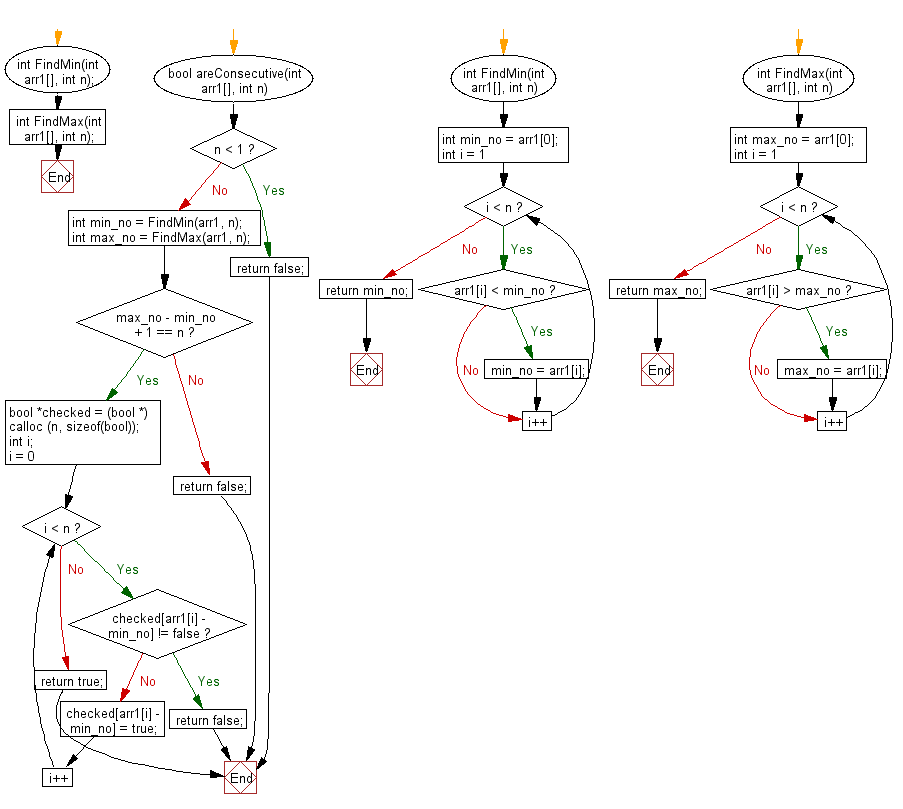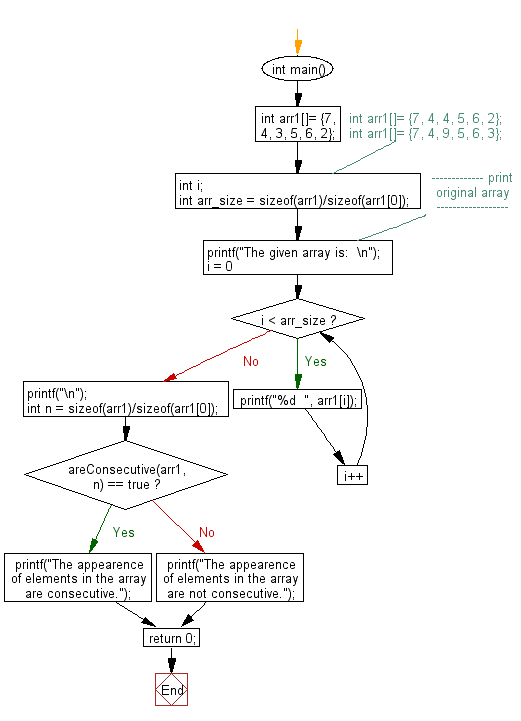﻿ C exercises: Checks whether the elements in an unsorted array appears consecutively or not - w3resource# C Exercises: Checks whether the elements in an unsorted array appears consecutively or not

## C Array: Exercise-92 with Solution

Write a program in C that checks whether the elements in an unsorted array appears consecutively or not.

Sample Solution:

C Code:

``````#include<stdio.h>
#include<stdlib.h>
#include <stdbool.h>

int FindMin(int arr1[], int n);
int FindMax(int arr1[], int n);

bool areConsecutive(int arr1[], int n)
{
if ( n <  1 )
return false;
int min_no = FindMin(arr1, n);
int max_no = FindMax(arr1, n);
if (max_no - min_no  + 1 == n)
{
bool *checked = (bool *) calloc (n, sizeof(bool));
int i;
for (i = 0; i < n; i++)
{
if ( checked[arr1[i] - min_no] != false )
return false;
checked[arr1[i] - min_no] = true;
}
return true;
}
return false;
}

int FindMin(int arr1[], int n)
{
int min_no = arr1;
for (int i = 1; i < n; i++)
if (arr1[i] < min_no)
min_no = arr1[i];
return min_no;
}

int FindMax(int arr1[], int n)
{
int max_no = arr1;
for (int i = 1; i < n; i++)
if (arr1[i] > max_no)
max_no = arr1[i];
return max_no;
}

int main()
{
int arr1[]= {7, 4, 3, 5, 6, 2};
//    int arr1[]= {7, 4, 4, 5, 6, 2};
//    int arr1[]= {7, 4, 9, 5, 6, 3};
int i;
int arr_size = sizeof(arr1)/sizeof(arr1);
//------------- print original array ------------------
printf("The given array is:  \n");
for(i = 0; i < arr_size; i++)
{
printf("%d  ", arr1[i]);
}
printf("\n");
//-----------------------------------------------------------
int n = sizeof(arr1)/sizeof(arr1);
if(areConsecutive(arr1, n) == true)
printf("The appearence of elements in the array are consecutive.");
else
printf("The appearence of elements in the array are not consecutive.");
return 0;
}
```
```

Sample Output:

```The given array is:
7  4  3  5  6  2
The appearence of elements in the array are consecutive.

The given array is:
7  4  4  5  6  2
The appearence of elements in the array are not consecutive.

The given array is:
7  4  9  5  6  3
The appearence of elements in the array are not consecutive.
```

Pictorial Presentation:Flowchart 1:Flowchart 2:C Programming Code Editor:

Improve this sample solution and post your code through Disqus.

﻿